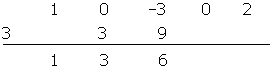Chapters

In order to explain the steps to implement Ruffini's rule, an example division will be used throughout the explaination:

(x4 − 3x² + 2 ) : (x − 3)

## 1

If the polynomial is not complete, complete it by adding the missing terms with zeros.

## 2

Set the coefficients of the dividend in one line.

## 3

In the bottom left, place the opposite of the independent term of the divisor.

## 4

Draw a line and lower the first coefficient.## 5

Multiply this coefficient by the divisor and place it under the following term.## 6## 7

Repeat the process above.Repeat the process:Repeat, again:## 8

The last number obtained, 56, is the remainder.

## 9

The quotient is a polynomial of lower degree and whose coefficients are the ones obtained in the division.

x³ + 3 x² + 6x +18

## Example

Divide by Ruffini's rule:

(x5 − 32) : (x − 2)C(x) = x4 + 2x³ + 4x² + 8x + 16

R = 0

Did you like the article?(1 votes, average: 5.00 out of 5)Loading...

Emma

I am passionate about travelling and currently live and work in Paris. I like to spend my time reading, gardening, running, learning languages and exploring new places.

Did you like
this resource?

Bravo!